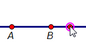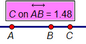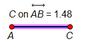﻿ Menus > Measure Menu > Value of Point > How to Construct a Slider

# How to Construct a SliderOften it is useful to construct a slider for controlling a numeric value. For example, if you wanted to create a graph of the line y = mx + b in which you can slide a point back and forth to control the values of m and b, you might want to set up sliders for m and b. To make a slider for m:

 1.Use the Line tool to construct a line. Label the two resulting points A and B.2.Use the Point tool to construct point C on the line.3.Select point C and choose 4.Select and hide the line and point B, leaving only two points and the measured ratio.5.Construct a segment connecting A and C.6.Double-click the measured ratio with the Text tool, and set its label to m. Hide point A, and change the label of point C to m to match the label of the value.

Your basic slider is complete. As you drag point m, the value changes accordingly.

You can vary this construction for a number of purposes.

For positive values, use a ray instead of a line in step 1.

For values between 0 and 1, use a segment instead of a line in step 1.

For a different scale, change the distance from A to B, or multiply the slider value by some scaling factor.

For integer values, use the Calculator to round the value of the slider.

You can also create a numeric value (a parameter) directly, using the command. The advantage of the slider described here is that sliding the control point back and forth provides a powerful, visual way to change the value.